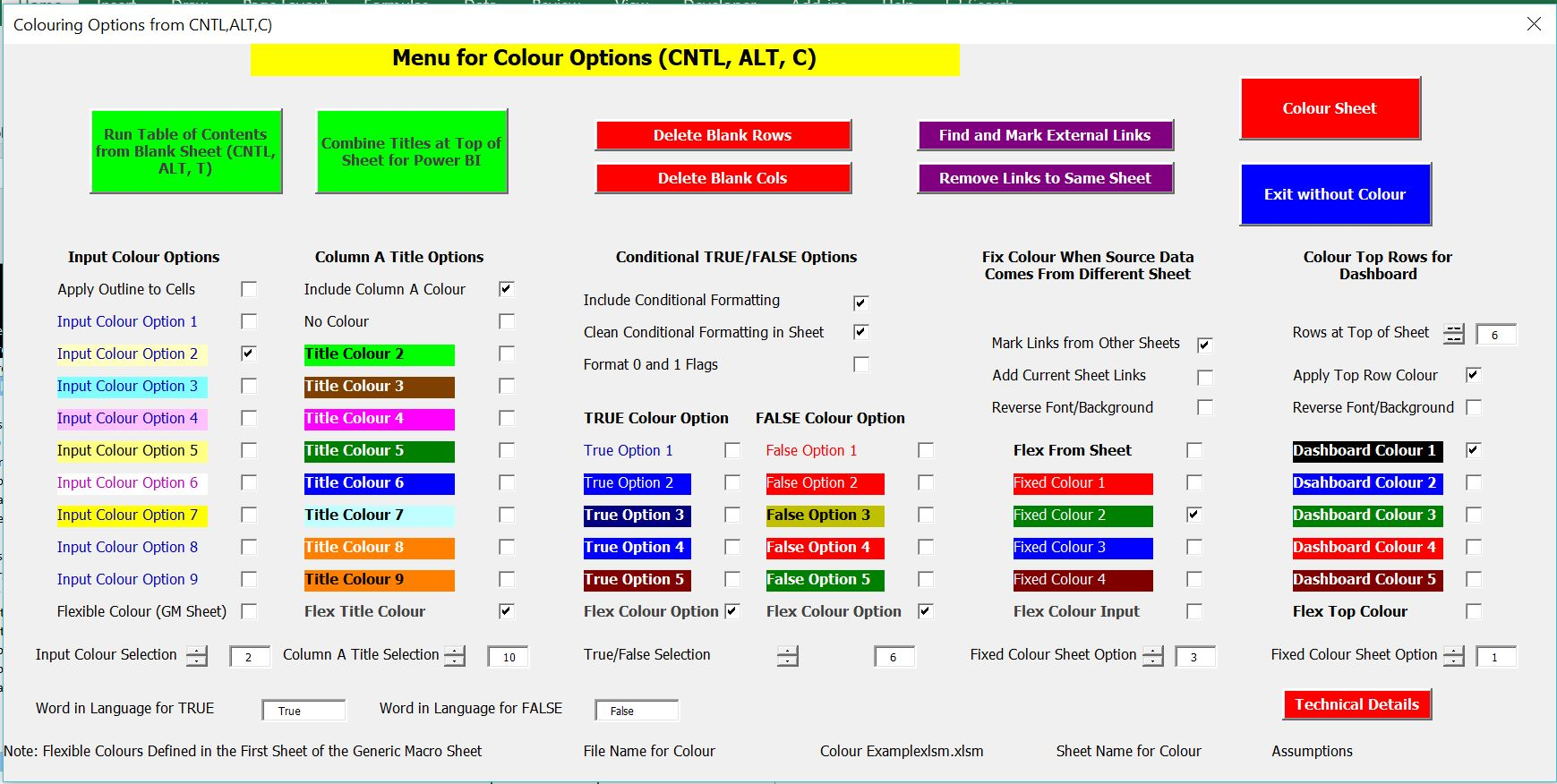# Sheet Name Remove

This page shows how to create a macro that automatically removes the sheet names of the current sheet in formulas of an excel spreadsheet. Through using a macro, you can take out the current sheet from all of the formulas in that use the current sheet name of your workbook. The method used is to get the sheet name with a function and then use the instring function to replace the sheet name with a function. The VBA code for running this program is shown in this page.

## Mechanics of Running the Macro if you Don’t Care About the VBA

If you refer to another sheet when typing in a formula in an excel sheet, the current sheet name will appear in formula. The current sheet name is of course not necessary for making the formula work. Further, the current sheet name  can be irritating and make the formulas difficult to read and interpret.  An example of the problem is shown on the screenshot below.

To remove the sheet links you can use the Generic Macros excel file. One of the buttons on the form that appears after pressing CNTL, ALT, C will scan your sheet and remove the names. The purple button that allows you to do this is shown in the screenshot below.## VBA Code for Removing Sheet Links

You could  VBA code can be copied into your sheet or your personal workbook if you want to use it. If you try this be careful wth the functions that find rows and columns of the sheet.  Note that instead of using the find function with worksheetfunction, the INSTR VBA function is used.  This function is a lot more stable then using the find function when there is an error.  The macro shown below works through cells in a sheet and replaces the sheet name with a blank. It allows for five sheet names in the formula.

.

```Sub x_replace_sheet_name()

Dim sheet_name_lenght As Single

x_find_rows ' run programs to find the number of rows to reduce the time
x_find_cols

Application.StatusBar = " Searching to Replace in " & max_row & " Rows and " & max_col & " Columns "

calculation_state = Application.Calculation
Application.Calculation = xlCalculationManual

sheet_name_active = ActiveSheet.Name ' get the current sheet name so you can look for this.

test_start = Left(sheet_name_active, 1) ' test_start is the leftmost character in the current sheet name

space_test = False ' When there is a space in the sheet name, then ""
For i = 1 To Len(sheet_name_active)
sheet_name_char = Mid(sheet_name_active, i, 1)
If sheet_name_char = " " Then space_test = True
Next i

If space_test Then ' put quotes around the sheet name
sheet_name_test = "'" & sheet_name_active & "'!"
Else
sheet_name_test = sheet_name_active & "!"
End If

sheet_name_length = Len(sheet_name_test)

msgtest = MsgBox(" Looking for sheet name " & sheet_name_test & " Is this ok ", vbOKCancel, " Sheet Name Replace Program")

If msgtest = 2 Then Exit Sub

For row = 1 To max_row
For col = 1 To max_col

Cells(row, col).Select
cell_string = Cells(row, col).Formula ' Only replace formulas
left_character = Left(cell_string, 1)
If left_character <> "=" Then GoTo continue1:

If cell_string = "" Then GoTo continue1: ' Skip over blanks

' Replace the cell string with blank

start_mid = 1

For j = 1 To 5

If start_mid = 0 Then GoTo continue2:

start_mid = InStr(start_mid, cell_string, sheet_name_test) ' key item that replaces

If start_mid = 0 Then GoTo continue2:

MsgBox " Trying to Replace " & cell_string & " Starting in " & start_mid & " Row " & row & " Column " & col

cell_string = WorksheetFunction.Replace(cell_string, start_mid, sheet_name_length, "") ' key item that replaces

On Error Resume Next
Cells(row, col).Formula = cell_string

continue2:
Next j
continue1:

Next col

Next row

Application.Calculation = calculation_state
Application.StatusBar = False

Range("A1").Select
'
Application.Goto Range("A1")

End Sub

.```